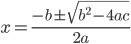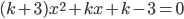# Discriminant

## Discriminant of quadratic polynomial

Time for investigation, almost 75 min Earlier in the course: Enough playing around with the graphs of quadratic functions & formula for zeros. Goal: Students find out which part of formula above influences the number of solutions of second order polynomial. This idea is simple, takes time for students to do and gives them opportunity to rehearse how to solve zeroes, how to express themselves mathematically. Students are asked to do following ( in small groups)
1. With Geogebra, find out second order polynomial, which has a) 2 b) 1 c) 0 zeros.
2. By hand, calculate the zeros and find out, which part of the formula influences the number of solutions.
3. Make your own theory. ( Formulate your theory so that it uses same letters as in the formula for zeros of second order polynomial. Write down your theory, send or show to teacher.
4. Use your theory, to find out for which k, equationhas 2 solutions, 1 solution or 0 solutions. You can use Geogebra to solve this. ( there are at least 2 possibilities to go, students use slider or CAS)